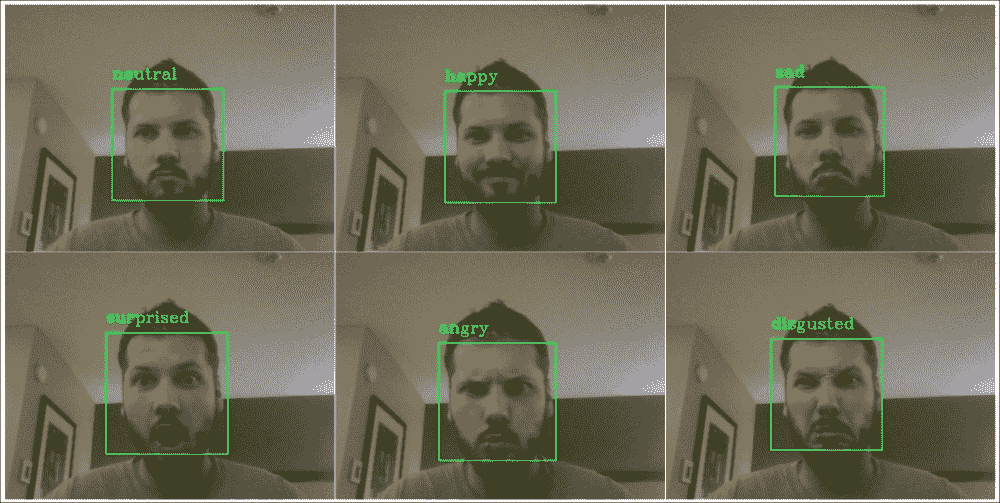# 七、学习识别面部表情

• 人脸检测：我们将使用 Viola 和 Jones 流行的 Haar 级联分类器，为此 OpenCV 提供了一系列预训练的样本。 我们将利用面部叶栅和眼睛叶栅来可靠地检测并对齐各个框架中的面部区域。
• 面部表情识别：我们将训练多层感知器，以识别每张检测到的面部中较早列出的六种不同的情绪表情。 这种方法的成功关键取决于我们组装的训练集，以及我们选择应用于该集中每个样本的预处理。 为了提高我们自记录训练集的质量，我们将确保使用仿射变换对齐所有数据样本，并通过应用减少特征空间的维数[ 主成分分析PCA）。 有时将所得表示形式也称为 EigenFace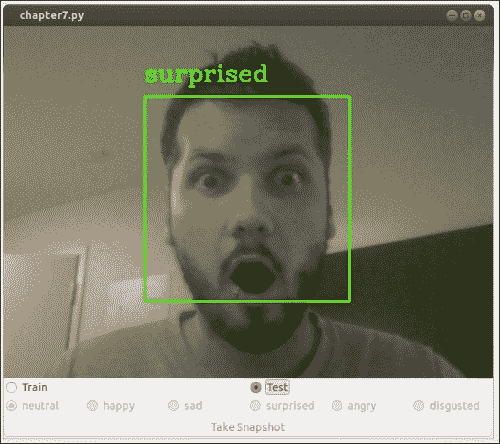# 规划应用

• chapter7：本章的主要脚本和入口点。
• chapter7.FaceLayout：基于gui.BaseLayout的自定义布局，可在两种不同模式下运行：
• 训练模式：在训练模式下，应用将收集图像帧，检测其中的脸部，根据面部表情分配标签，并在退出时将所有收集的数据样本保存在文件中，以便可以通过解析datasets.homebrew
• 测试模式：在测试模式下，应用将检测每个视频帧中的人脸，并使用预训练的 MLP 预测相应的类别标签。
• chapter3.main：启动 GUI 应用的main函数例程。
• detectors.FaceDetector：用于面部检测的类。
• detect：一种用于检测灰度图像中人脸的方法。 可选地，将图像缩小以提高可靠性。 成功检测后，该方法将返回提取的头部区域。
• align_head：一种使用仿射变换对提取的头部区域进行预处理的方法，以使生成的脸部居中并直立。
• classifiers.Classifier：一个抽象基类，它定义所有分类器的公共接口（与第 6 章，“学习识别交通标志”中的相同）。
• classifiers.MultiLayerPerceptron：通过使用以下公共方法来实现 MLP 的类：
• fit：一种将 MLP 拟合到训练数据的方法。 它以训练数据矩阵作为输入，其中每行是训练样本，列包含特征值和标签向量。
• evaluate：一种通过在训练后将 MLP 应用于某些测试数据来评估 MLP 的方法。 它以测试数据矩阵作为输入，其中每行是测试样本，每列包含特征值和标签向量。 该函数返回三种不同的表现指标：准确率，准确率和召回率。
• predict：一种预测某些测试数据的类标签的方法。 我们向用户公开此方法，以便可以将其应用于任意数量的数据样本，这在测试模式下非常有用，当我们不想评估整个数据集而只预测单个数据样本的标签时 。
• save：一种将经过训练的 MLP 保存到文件的方法。
• load：一种从文件加载预训练的 MLP 的方法。
• train_test_mlp：通过将 MLP 应用于我们的自记录数据集来训练和测试 MLP 的脚本。 该脚本将探索不同的网络架构，并将具有最佳泛化表现的网络架构存储在文件中，以便可以在以后加载经过预先​​训练的分类器。
• datasets.homebrew：解析自记录训练集的类。 与上一章类似，该类包含以下方法：
• load_data：一种加载训练集，通过extract_features函数对其执行 PCA 并将数据拆分为训练集和测试集的方法。 可选地，可以将预处理后的数据存储在文件中，这样我们以后就可以加载它，而不必再次解析数据。
• load_from_file：一种加载先前存储的预处理数据集的方法。
• extract_features：一种提取所选特征的方法（在本章中：对数据执行 PCA）。 我们将此函数公开给用户，以便可以将其应用于任意数量的数据样本，这在测试模式下非常有用，当我们不想解析整个数据集而只预测单个数据样本的标签时。
• gui：一个模块，提供一个 wxPython GUI 应用来访问捕获设备并显示视频提要。 这与我们在前面各章中使用的模块相同。
• gui.BaseLayout：可以从中构建更复杂布局的通用布局。 本章不需要对基本布局进行任何修改。

# 人脸检测

OpenCV 预装了系列复杂分类器，用于通用对象检测。 也许，最广为人知的检测器是用于面部检测的基于 Haar 级联的特征检测器，这是由 Paul Viola 和 Michael Jones 发明的。

## 基于 Haar 级联的分类器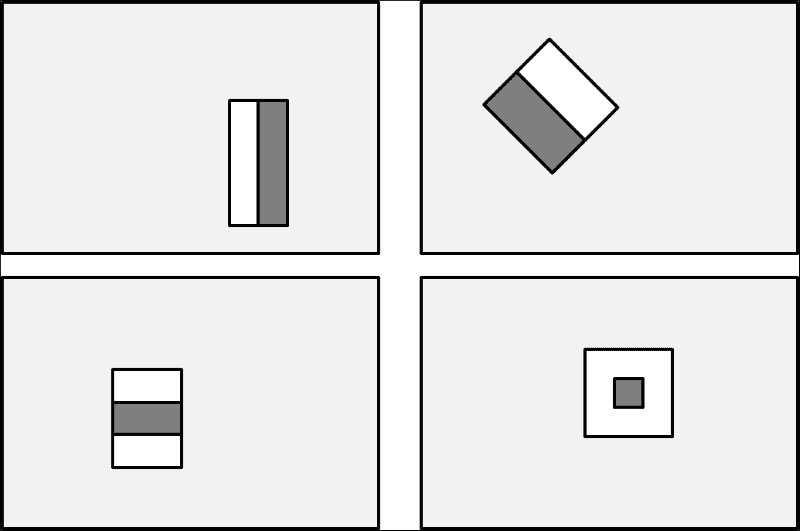## 使用预训练的级联分类器

import cv2

faces = face_casc.detectMultiScale(frame, scaleFactor=1.1,
minNeighbors=3)


detectMultiScale函数带有许多选项：

• minFeatureSize：要考虑的最小脸部尺寸（例如 20×20 像素）。
• searchScaleFactor：要重新缩放图像的比例（缩放金字塔）。 例如，值 1.1 会逐渐将输入图像的尺寸减小 10%，从而比更大的值更有可能找到人脸。
• minNeighbors：每个候选矩形必须保留的邻居数。 通常，选择 3 或 5。
• flags：旧级联的选项（较新的级联将忽略）。 例如，要查找所有面孔还是仅查找最大面孔（cv2.cv.CASCADE_FIND_BIGGEST_OBJECT）。

for (x, y, w, h) in faces:
# draw bounding box on frame
cv2.rectangle(frame, (x, y), (x + w, y + h), (100, 255, 0), 2)


## FaceDetector类

import cv2
import numpy as np

class FaceDetector:
def __init__(


        self.face_casc = cv2.CascadeClassifier(face_casc)
if self.face_casc.empty():
face_casc
raise SystemExit


        self.left_eye_casc = cv2.CascadeClassifier(left_eye_casc)
if self.left_eye_casc.empty():
raise SystemExit
self.right_eye_casc =
if self.right_eye_casc.empty():
raise SystemExit


self.scale_factor = scale_factor


### 在灰度图像中检测人脸

def detect(self, frame):
frameCasc = cv2.cvtColor(cv2.resize(frame, (0, 0), fx=1.0 / self.scale_factor, fy=1.0 / self.scale_factor), cv2.COLOR_RGB2GRAY)
faces = self.face_casc.detectMultiScale(frameCasc,
scaleFactor=1.1, minNeighbors=3,
flags=cv2.cv.CV_HAAR_FIND_BIGGEST_OBJECT) * self.scale_factor


for (x, y, w, h) in faces:
head = cv2.cvtColor(frame[y:y + h, x:x + w], cv2.COLOR_RGB2GRAY)


cv2.rectangle(frame, (x, y), (x + w, y + h), (100, 255, 0), 2)


return True, frame, head


return False, frame, None


### 预处理检测到的脸部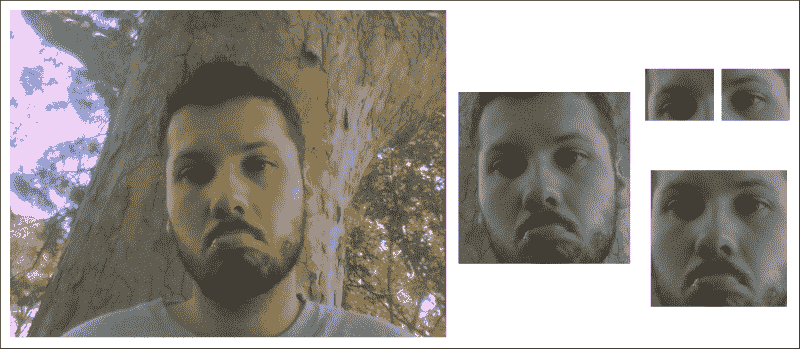def align_head(self, head):


FaceDetector构造器中的文件加载这些眼睛级联后，将它们应用于输入图像（head）：

    left_eye_region = head[0.2*height:0.5*height, 0.1*width:0.5*width]
left_eye = self.left_eye_casc.detectMultiScale(left_eye_region, scaleFactor=1.1, minNeighbors=3, flags=cv2.cv.CV_HAAR_FIND_BIGGEST_OBJECT)


left_eye_center = None
for (xl, yl, wl, hl) in left_eye:
# find the center of the detected eye region
left_eye_center = np.array([0.1 * width + xl + wl / 2, 0.2 * height + yl + hl / 2])
break # need only look at first, largest eye


right_eye_region = head[0.2*height:0.5*height, 0.5*width:0.9*width]
right_eye = self.right_eye_casc.detectMultiScale(right_eye_region, scaleFactor=1.1, minNeighbors=3,flags=cv2.cv.CV_HAAR_FIND_BIGGEST_OBJECT)
right_eye_center = None
for (xr, yr, wr, hr) in right_eye:
# find the center of the detected eye region
right_eye_center = np.array([0.5 * width + xr + wr / 2, 0.2 * height + yr + hr / 2])
break  # need only look at first, largest eye


if left_eye_center is None or right_eye_center is None:


desired_eye_x = 0.25
desired_eye_y = 0.2
desired_img_width = 200
desired_img_height = desired_img_width


eye_center = (left_eye_center + right_eye_center) / 2
eye_angle_deg = np.arctan2(
right_eye_center – left_eye_center,
right_eye_center – left_eye_center) *
180.0 / cv2.cv.CV_PI


eye_size_scale = (1.0 - desired_eye_x * 2) * desired_img_width / np.linalg.norm(right_eye_center – left_eye_center)


rot_mat = cv2.getRotationMatrix2D(tuple(eye_center), eye_angle_deg, eye_size_scale)


rot_mat[0,2] += desired_img_width*0.5 – eye_center
rot_mat[1,2] += desired_eye_y*desired_img_height – eye_center


res = cv2.warpAffine(head, rot_mat, (desired_img_width, desired_img_height))
return True, res


# 面部表情识别

1. 在训练模式下加载chapter7.py GUI，以组装训练集。
2. 通过train_test_mlp.py在训练集上训练 MLP 分类器。 由于此步骤可能需要很长时间，因此该过程将以其自己的脚本进行。 成功训练后，将训练后的权重存储在文件中，以便我们可以在下一步中加载预训练的 MLP。
3. 在测试模式下加载chapter7.py GUI，以实时对实时视频流上的面部表情进行分类。 此步骤涉及加载几个预训练的级联分类器以及我们的预训练的 MLP 分类器。 然后，这些分类器将应用于每个捕获的视频帧。

## 组装训练套件

GUI 将为用户提供记录以下六个情感表达之一的选项：中立，快乐，悲伤，惊讶，生气和恶心。 单击按钮后，该应用将对检测到的面部区域进行快照，并在退出时将所有收集的数据样本存储在文件中。 然后，可以从文件中加载这些样本，并将其用于训练train_test_mlp.py中的 MLP 分类器，如先前在第二步中所述。

### 运行屏幕截图

import time
import wx
from os import path
import cPickle as pickle

import cv2
import numpy as np

from datasets import homebrew
from detectors import FaceDetector
from classifiers import MultiLayerPerceptron
from gui import BaseLayout

def main():
capture = cv2.VideoCapture(0)
if not(capture.isOpened()):
capture.open()

capture.set(cv2.cv.CV_CAP_PROP_FRAME_WIDTH, 640)
capture.set(cv2.cv.CV_CAP_PROP_FRAME_HEIGHT, 480)

# start graphical user interface
app = wx.App()
layout = FaceLayout(None, -1, 'Facial Expression Recognition', capture)
layout.init_algorithm()
layout.Show(True)
app.MainLoop()

if __name__ == '__main__':
main()


### GUI 构造器

class FaceLayout(BaseLayout):


    def _init_custom_layout(self):
# initialize data structure
self.samples = []
self.labels = []

# call method to save data upon exiting
self.Bind(wx.EVT_CLOSE, self._on_exit)


    def init_algorithm(


        self.dataFile = save_training_file


        self.faces = FaceDetector(face_casc, left_eye_casc, right_eye_casc)


        # load preprocessed dataset to access labels and PCA
# params
self.all_labels = np.unique(np.hstack((y_train,
y_test)))

self.MLP = MultiLayerPerceptron( np.array([self.pca_V.shape, len(self.all_labels)]), self.all_labels)


            else:
print "Warning: Testing is disabled"
print "Could not find pre-trained MLP file ",
self.testing.Disable()
else:
print "Warning: Testing is disabled"
print "Could not find preprocessed data file ", loadPreprocessedData
self.testing.Disable()


### GUI 布局

def _create_custom_layout(self):
# create horizontal layout with train/test buttons
pnl1 = wx.Panel(self, -1)
self.training = wx.RadioButton(pnl1, -1, 'Train', (10, 10), style=wx.RB_GROUP)
hbox1 = wx.BoxSizer(wx.HORIZONTAL)
pnl1.SetSizer(hbox1)


self.Bind(wx.EVT_RADIOBUTTON, self._on_training, self.training)


# create a horizontal layout with all buttons
pnl2 = wx.Panel(self, -1 )
self.neutral = wx.RadioButton(pnl2, -1, 'neutral', (10, 10), style=wx.RB_GROUP)
hbox2 = wx.BoxSizer(wx.HORIZONTAL)

pnl2.SetSizer(hbox2)


pnl3 = wx.Panel(self, -1)
self.snapshot = wx.Button(pnl3, -1, 'Take Snapshot')
self.Bind(wx.EVT_BUTTON, self.OnSnapshot, self.snapshot)
hbox3 = wx.BoxSizer(wx.HORIZONTAL)
pnl3.SetSizer(hbox3)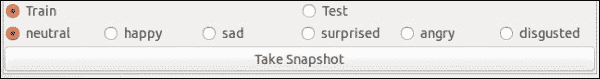# display the button layout beneath the video stream
self.panels_vertical.Add (pnl1, flag=wx.EXPAND | wx.TOP, border=1)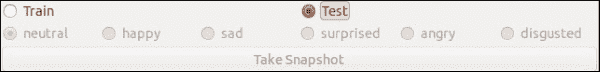def _on_testing(self, evt):
"""Whenever testing mode is selected, disable all training-related buttons"""
self.neutral.Disable()
self.happy.Disable()
self.surprised.Disable()
self.angry.Disable()
self.disgusted.Disable()
self.snapshot.Disable()


def _on_training(self, evt):
"""Whenever training mode is selected, enable all
training-related buttons"""
self.neutral.Enable()
self.happy.Enable()
self.surprised.Enable()
self.angry.Enable()
self.disgusted.Enable()
self.snapshot.Enable()


### 处理当前帧

def _process_frame(self, frame):


    return frame


### 将训练样本添加到训练集中

def _on_snapshot(self, evt):
if self.neutral.GetValue():
label = 'neutral'
elif self.happy.GetValue():
label = 'happy'
elif self.surprised.GetValue():
label = 'surprised'
elif self.angry.GetValue():
label = 'angry'
elif self.disgusted.GetValue():
label = 'disgusted'


    if self.head is None:
print "No face detected"
else:


if success:
print "Added sample to training set"
self.labels.append(label)
else:
print "Could not align head (eye detection failed?)"


### 将完整的训练集转储到文件中

def _on_exit(self, evt):
"""Called whenever window is closed"""
# if we have collected some samples, dump them to file
if len(self.samples) > 0:


    # make sure we don't overwrite an existing file
if path.isfile(self.data_file):
filename, fileext = path.splitext(self.data_file)
offset = 0
while True: # a do while loop
file = filename + "-" + str(offset) + fileext
if path.isfile(file):
offset += 1
else:
break
self.data_file = file


    f = open(self.dataFile, 'wb')
pickle.dump(self.samples, f)
pickle.dump(self.labels, f)
f.close()


    print "Saved", len(self.samples), "samples to", self.data_file
self.Destroy()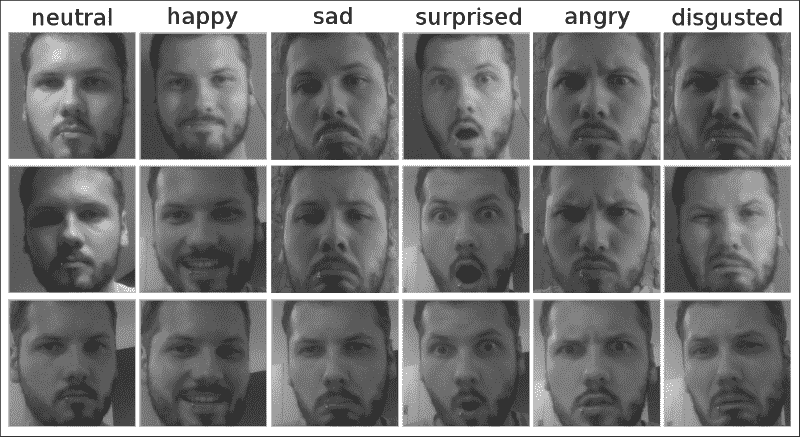## 特征提取

### 预处理数据集

import cv2
import numpy as np

import csv
from matplotlib import cm
from matplotlib import pyplot as plt

from os import path
import cPickle as pickle

save_to_file=None, plot_samples=False, seed=113):


# prepare lists for samples and labels
X = []
labels = []
print "Could not find file", load_from_file
return (X, labels), (X, labels), None, None
else:


# perform feature extraction
# returns preprocessed samples, PCA basis vectors & mean
X, V, m = extract_features(samples, num_components=num_components)


# shuffle dataset
np.random.seed(seed)
np.random.shuffle(X)
np.random.seed(seed)
np.random.shuffle(labels)

# split data according to test_split
X_train = X[:int(len(X)*(1-test_split))]
y_train = labels[:int(len(X)*(1-test_split))]

X_test = X[int(len(X)*(1-test_split)):]
y_test = labels[int(len(X)*(1-test_split)):]


if save_to_file is not None:
# dump all relevant data structures to file
f = open(save_to_file, 'wb')
pickle.dump(X_train, f)
pickle.dump(y_train, f)
pickle.dump(X_test, f)
pickle.dump(y_test, f)
pickle.dump(V, f)
pickle.dump(m, f)
f.close()
print "Save preprocessed data to", save_to_file


return (X_train, y_train), (X_test, y_test), V, m


### 主成分分析

PCA 是一种降维缩减技术，在处理高维数据时会很有用。 从某种意义上讲，您可以将图像视为高维空间中的一个点。 如果将高度为m和宽度为n的 2D 图像展平（通过合并所有行或所有列），我们将获得长度为m × n的（特征）向量。 该向量中的第i个元素的值是图像中第i个像素的灰度值。 为了描述具有这些确切尺寸的每个可能的 2D 灰度图像，我们将需要一个m × n维向量空间，其中包含 256 个提升为m × n向量的幂的向量。 哇！ 考虑这些数字时想到的一个有趣的问题如下：是否可以有一个较小，更紧凑的向量空间（使用少于m × n特征）来很好地描述所有这些图像？ 毕竟，我们之前已经意识到灰度值并不是内容的最有用信息。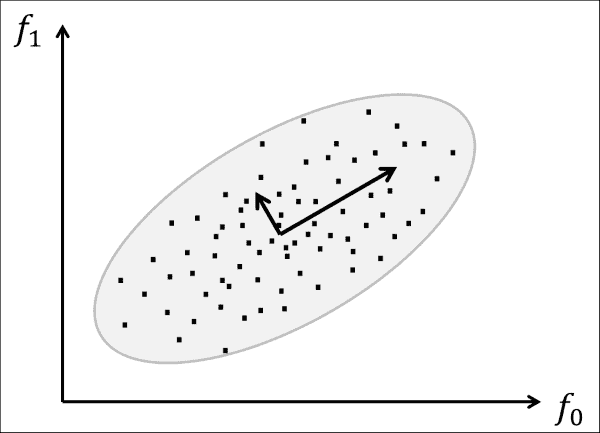PCA 的作用是旋转所有数据点，直到数据与两个（两个插图向量）对齐，这两个轴解释了大多数扩散的数据。 PCA 认为这两个轴是最有用的，因为如果沿着它们走动，可以看到大多数数据点是分开的。 用更专业的术语来说，PCA 旨在通过正交线性变换将数据变换为新的坐标系。 选择新坐标系时，如果将数据投影到这些新轴上，则第一个坐标（称为第一主成分）观察到最大方差。 在前面的图像中，绘制的小向量与协方差矩阵的特征向量相对应，这些向量发生了偏移，因此它们的尾部位于分布的均值处。

def extract_feature(X, V=None, m=None, num_components=50):


    if V is None or m is None:
# need to perform PCA from scratch
if num_components is None:
num_components = 50

# cols are pixels, rows are frames
Xarr = np.squeeze(np.array(X).astype(np.float32))

# perform PCA, returns mean and basis vectors
m, V = cv2.PCACompute(Xarr)


PCA 的优点在于，根据定义，第一个主要成分解释了大部分数据差异。 换句话说，第一个主成分是数据的最有用的信息。 这意味着我们不需要保留所有组件就可以很好地表示数据！ 我们可以将自己限制在num_components信息最丰富的国家：

    # use only the first num_components principal components
V = V[:num_components]


    for i in xrange(len(X)):
X[i] = np.dot(V, X[i] - m[0, i])

return X, V, m


## 多层感知器

### 感知器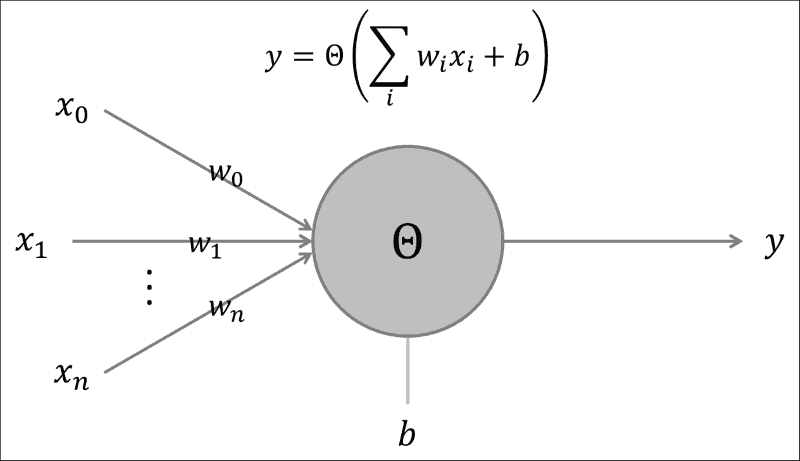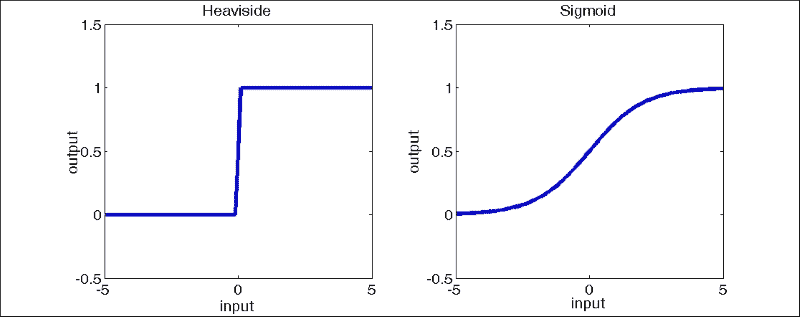### 深度架构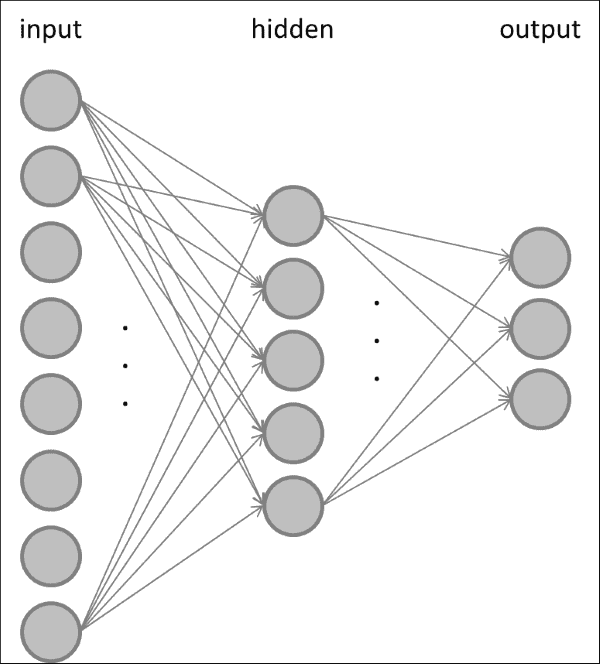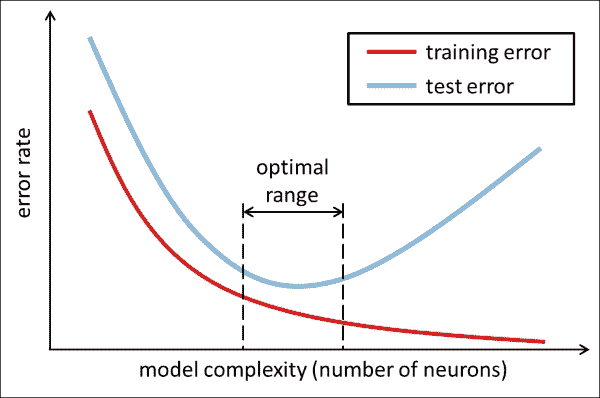MLP 通过调整其权重进行学习，以便在显示类别为c的输入样本时，输出层中第c个节点处于活动状态，而其他所有节点均处于静默状态。 MLP 通过反向传播进行训练，这是一种计算损失函数相对于神经突触权重或神经元偏置的偏导数的算法。 网络。 然后，这些偏导数可用于更新网络中的权重和偏差，以逐步减少总体损失。

## 用于面部表情识别的 MLP

from abc import ABCMeta, abstractmethod

class Classifier:
"""Abstract base class for all classifiers"""
__metaclass__ = ABCMeta

@abstractmethod
def fit(self, X_train, y_train):
pass

@abstractmethod
def evaluate(self, X_test, y_test, visualize=False):
pass


class MultiLayerPerceptron(Classifier):


    def __init__(self, layer_sizes, class_labels, params=None):
self.num_features = layer_sizes
self.num_classes = layer_sizes[-1]
self.class_labels = class_labels
self.params = params or dict()


        self.model = cv2.ANN_MLP()
self.model.create(layer_sizes)


def _labels_str_to_num(self, labels):
""" convert string labels to their corresponding ints """
return np.array([int(np.where(self.class_labels == l)) for l in labels])

def _labels_num_to_str(self, labels):
"""Convert integer labels to their corresponding string names """
return self.class_labels[labels]


def load(self, file):
""" load a pre-trained MLP from file """

def save(self, file):
""" save a trained MLP to file """
self.model.save(file)


### 训练 MLP

def fit(self, X_train, y_train, params=None):
""" fit model to data """
if params is None:
params = self.params


params = dict(
term_crit = (cv2.TERM_CRITERIA_COUNT, 300, 0.01), train_method = cv2.ANN_MLP_TRAIN_PARAMS_BACKPROP)


y_train = self._labels_str_to_num(y_train)
y_train = self._one_hot(y_train).reshape(-1, self.num_classes)
self.model.train(X_train, y_train, None, params=params)


_one_hot中处理了单热代码：

def _one_hot(self, y_train):
"""Convert a list of labels into one-hot code """


    num_samples = len(y_train)
new_responses = np.zeros(num_samples * self.num_classes, np.float32)


    resp_idx = np.int32(y_train +
np.arange(num_samples)  self.num_classes)


    new_responses[resp_idx] = 1
return new_responses


### 测试 MLP

def evaluate(self, X_test, y_test, visualize=False):
""" evaluate model performance """


    ret, Y_vote = self.model.predict(X_test)
y_test = self._labels_str_to_num(y_test)
accuracy = self._accuracy(y_test, Y_vote)
precision = self._precision(y_test, Y_vote)
recall = self._recall(y_test, Y_vote)

return (accuracy, precision, recall)


def predict(self, X_test):
""" predict the labels of test data """
ret, Y_vote = self.model.predict(X_test)

# find the most active cell in the output layer
y_hat = np.argmax(Y_vote, 1)

# return string labels
return self._labels_num_to_str(y_hat)


### 运行脚本

import cv2
import numpy as np

from datasets import homebrew
from classifiers import MultiLayerPerceptron

def main():
# training data can be recorded using chapter7.py in training
# mode
(X_train, y_train),(X_test, y_test) =
if len(X_train) == 0 or len(X_test) == 0:
print "Empty data"
raise SystemExit

# convert to numpy
X_train = np.squeeze(np.array(X_train)).astype(np.float32)
y_train = np.array(y_train)
X_test = np.squeeze(np.array(X_test)).astype(np.float32)
y_test = np.array(y_test)

# find all class labels
labels = np.unique(np.hstack((y_train, y_test)))


    params = dict( term_crit = (cv2.TERM_CRITERIA_COUNT, 300, 0.01), train_method=cv2.ANN_MLP_TRAIN_PARAMS_BACKPROP, bp_dw_scale=0.001, bp_moment_scale=0.9 )


    save_file = 'params/mlp.xml'
num_features = len(X_train)
num_classes = len(labels)

# find best MLP configuration
print "1-hidden layer networks"
best_acc = 0.0 # keep track of best accuracy
for l1 in xrange(10):
# gradually increase the hidden-layer size
layer_sizes = np.int32([num_features,
(l1 + 1) * num_features / 5,
num_classes])
MLP = MultiLayerPerceptron(layer_sizes, labels)
print layer_sizes


MLP 在X_train上受过训练，并在X_test上进行过测试：

    MLP.fit(X_train, y_train, params=params)
(acc, _, _) = MLP.evaluate(X_train, y_train)
print " - train acc = ", acc
(acc, _, _) = MLP.evaluate(X_test, y_test)
print " - test acc = ", acc


    if acc > best_acc:
# save best MLP configuration to file
MLP.save(saveFile)
best_acc = acc


# 全部放在一起

def _process_frame(self, frame):
""" detects face, predicts face label in testing mode """


    success, frame, self.head = self.faces.detect(frame)


    # in testing mode: predict label of facial expression
if success and self.testing.GetValue():


    # if face found: preprocess (align)
if success:
# extract features using PCA (loaded from file)
X, _, _ = homebrew.extract_features([head.flatten()], self.pca_V, self.pca_m)


    # predict label with pre-trained MLP
label = self.MLP.predict(np.array(X))


    # draw label above bounding box
cv2.putText(frame, str(label), (x,y-20), cv2.FONT_HERSHEY_COMPLEX, 1, (0,255,0), 2)
break # need only look at first, largest face


    return frame